# Deep Neural Networks: First step towards thinking like Humans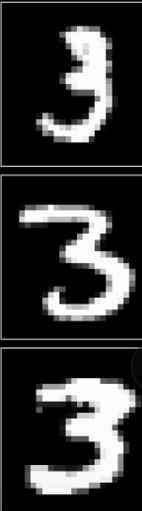Different images of digit 3 in different shapes and pixel value arrangements.

Let’s start with a simple image of the digit 3. The digit 3 may be written at various angles or directions, even at an extremely low resolution of 28 by 28 pixels, our brain has no trouble recognizing it as a three. Isn’t it intriguing that human brains can do this so effortlessly?

In all the three images above, even though the pixel value of each image is different from another, it is very easy to recognize each one. The light-sensitive cells, i.e. the rods and the cone cells, present in our eyes that light up when we see the images above are distinct from one another. But, if I told you to write a program that takes in a grid of 28 by 28 pixels like the one above, processes the image and finds out which digit it is, and gives us a number between 0 and 10, then it would be a dauntingly difficult task.

Machines are inherently dumb (as of now, at least), so, to make them smarter we induce some sort of intelligence so that they are capable of making a decision independently. This intelligence is explicitly programmed, say, with a list of if-else conditions. But what if we need to introduce intelligence into a machine without explicitly programming everything into it? Something that enables the machine to learn on its own? That’s where Machine Learning comes into play.

“ Machine learning can be defined as the process of inducing intelligence into a system or machine without explicit programming.”
- Andrew Ng, Stanford Professor.

The human body is the most intelligently engineered creation of the Almighty, and on it rests the most complex structure in the world, “Our Brain”. The powerhouse of our brain are the swift neurons. They are the ones running the whole system, from sensing any situation, to producing the appropriate response to it. According to the MIT Technology Review, human reaction time shows that the brain processes data no faster than 60 bits per second which is about 30 times faster than the best supercomputers. The human body has, time and again, inspired innovations and new technologies, one of which has been the most revolutionary, the Neural Networks. The idea behind neural networks is inspired from neurons that are the building blocks of our brain.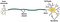https://sites.duke.edu/apep/module-2-the-abcs-of-intoxication/biology-and-chemistry-connections/neuron-structure-and-function/

The dendrites in the neuron receive signals via, either the sensors or, from other neurons which act as input to the processor(brain). Now, via a series of action potentials in the neuron itself, the signal passes through the whole neuron(Axon) and then reaches the end, which happens to be, the terminals of the Axon where with the help of a neurotransmitter the signal is balanced and passed onto the other neurons for further processing.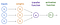Perceptron

Now, to explain the perceptron which is the functional unit of an artificial neural network; just as the biological neuron is to the biological neural network, we will take a daily life example which most of us happen to come across while in a market. So, how do we figure out if an apple is ripe or not?
Color, firmness, smell, luster are the features that we consider and then we come to a decision. Now, here come our brain and its neurons which help us take the decision on the basis of the inputs received via sensors like nose, eyes, etc. Now, let’s explore the same process but for a perceptron.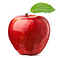https://ceklog.kindel.com/2013/02/19/why-nobody-can-copy-apple/

The inputs mentioned in the above paragraph are the features provided, i.e. color, firmness, smell, luster. For example that can be represented in the form of an array as follows: [Light red, good, Lemony, shiny]

Now, these are categorical attributes, later for processing, we will need to convert these into numerical values as in a perceptron the predictions are made via some scalar multiplications and additions.

Next are the weights which are multiplied to the inputs to show or state the importance of each feature of the fruit. For example, the fruit color here is more important than other features. So, it will be given more weight value. In both artificial and biological neural networks, a neuron does not just give out the value it receives from the transfer function. Instead, the activation function which is analogous to the rate of action potential firing in the brain takes the transfer function output and then transforms it once more before finally giving it as an output. The bias neuron is a special neuron added to each layer in the neural network, which simply stores the value of 1. Without a bias neuron, each neuron takes the input and multiplies it by the weight, with nothing else added to the equation. For example, it is not possible to input a value of 0 and output 2. In many cases, it is necessary to move the entire activation function to the left or right of the graph to generate the required output value which is made possible by the bias. Now the transfer function will combine all the outputs from the above-said scalar multiplications to generate a sum which we can say is the single value representing the quality of one apple. The transfer function can be written as:

z1 = w1*x1 + w2*x2 + b1

The sigmoid function is the oldest and most popular activation function. It is defined as follows:

σ(z1)=1/(1+e^-z1)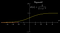Sigmoid function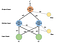This is how a transfer function and activation function works for a single perceptron

# But why do we need activation functions at all?

The reason is that the weighted sum is linear with respect to its inputs, i.e. it has a flat dependence on each of the inputs. In contrast, non-linear activation functions greatly expand our capacity to model curved functions and other complex patterns from our given data which are simply not possible with the linear functions. When comparing with the biological neural network that is in our brains, the activation function is at the end deciding what is to be fired to the next neuron.

# A Multilayer Perceptron or a Deep Neural Network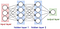A deep neural network

A simplified version of Deep Neural Network is represented as a hierarchical (layered) organization of neurons with connections to other neurons between any two layers. These neurons pass a message or signal to other neurons based on the received input from the previous neuron layer and form a complex network that learns with a feedback mechanism. The above given figure represents an ’N’ layered Deep Neural Network.

Let’s take the example of recognizing digits which we were talking about earlier, when I say neuron it specifically holds a number between 0 & 1 and not more than that. For example, the network starts with a bunch of neurons corresponding to each of the 28 x 28 pixels of the input image which is 784 neurons stacked on one another for the first layer which is the input and in total each one of these holds a number that represents the grayscale(having only one channel of color) value of the corresponding pixel ranging from 0 for black pixels up to 1 for white pixels. But before jumping into any further math for how one layer influences the next layer, let’s first figure out why it’s even necessary to have a layered structure to give intelligent results similar to our brain?
In this network, we have two hidden layers each one with any number of neurons and which is kind of arbitrary for now. The activations from one layer bring about activations in the next layer which is quite analogous to how in biological neural networks of neurons some groups of neurons firing cause others to fire. Finally, after all the activations brightest neuron of that output layer is the answer to what the digit in the given image represents.

When we break down to the simplest parts of the image, it will be the edges that make up the digits, so we can say that the second layer of the neural network picks up the various edges of the digits being fed into the input or the first layer and this way the next layer neurons identify more complex patterns like loops and lines from the previous layers, thereby finally lighting up the appropriate neuron for a given digit in the output layer.

Now let us get into the details of the whole process of passing one message or signal from the neuron in one layer to that of another:

The neurons in the second layer to pick up the edges from the input images as already mentioned above. We are going to assign a weight to each one of the connections between neurons from the first layer and the neurons from the first layer. As already said in the perceptron model above, the weights are just numbers that are multiplied with the numerical values in each neuron from the first layer and their weighted sum is computed. Now the weighted sum that is computed, we want it to be some value between 0 & 1, so the thing we want to do is to input this weighted sum into some function that squishes the real number line into the range between 0 & 1 and there comes the job of an activation function, here we are taking the popular function that does this is called the sigmoid function also known as a logistic curve where very negative inputs end up close to zero, very positive inputs end up close to 1 and just steadily increases around the input 0. So the activation of the neuron here is a measure of how positive the weighted sum is. Sometimes maybe we don’t want the neuron to light up when the weighted sum is bigger than 0 and want it to be active when the sum is bigger than say 10 and that is where we want some bias for it to be inactive before plugging it through the sigmoid squishification function. So basically the weights tell us what pixel pattern this neuron in the second layer is picking up on and the bias tells us how high the weighted sum needs to be for the neuron to be active. And that is just one neuron. Every other neuron in the next layer is going to be connected to all input pixel neurons from the first layer and each one of those input neuron connections has its weight associated with it also each one has some bias some other number that you add on to the weighted sum before squishing it with the sigmoid. This network has many such weights and biases that can be tweaked and turned to make this network behave in different ways. The weights and biases are initialized randomly.

# Forward Propagation

What we discussed above was all about the parameters involved in making the network learn, but now we are going to dive into the actual process of learning:

Forward propagation is the process in which the input data is fed in the forward direction through the network. Each hidden layer then accepts the input data, processes it as per the activation function, and passes it to the next layer. But in order to generate some output, the input data should be fed in the forward direction only. The data should not flow in the reverse direction during output generation otherwise it would form a cycle and the output will never be generated. And such network configurations are known as feed-forward neural networks which help in forward propagation.

So first we calculate the weighted sum of inputs(pre-activation) and then pass it through the activation function (activation) to give it a real value which fires the neurons in the next layers according to the bias added.
For example at the first node of the hidden layer, z1(pre-activation) is calculated first and then a1(activation) is calculated.

z1 is a weighted sum of inputs. Here, the weights are randomly generated.

z1 = w1*x1 + w2*x2 + b1 and a1 is the value of activation function applied on z1(a1 = σ(1/(1+e^-z1) )

Similarly, z2 = w3*x1 + w4*x2 + b2 and a2 is the value of activation function applied on z2(a2 = σ(1/(1+e^-z2)).

For any layer after the first hidden layer, the input of the next layer is the output from the previous layer.

z3 = w5*x1 + w6*x2 + b3 and a3 is the value of activation function applied on z3(a3 = σ(1/(1+e^-z3)).

# Backward Propagation

Now, due to the random initialization of the weights and biases of this network what we do is define a cost function as a way of telling the computer: “Bad choice!”, that output should have activations which are zero for most neurons, but one for the neuron we are trying to predict.
Mathematically what we do is add up the squares of the differences between each of those wrong output activations and the value that we want them to have and this is called the cost of a single training example.

We notice that this sum is small when the network confidently classifies the image correctly but it’s large when the network seems like it doesn’t know what it’s doing. So then we consider the average cost over all of the training examples. This average cost is now our measure of how bad or good the network is and how much more training and learning it needs.

The cost function here takes into consideration the network’s behavior overall training data examples. Now, tell me if you were a teacher, then does only showing a student their bad performance is enough or you should also guide the student for improving their performance by changing his studying or learning tactics? The second one also right? Similarly, we also need to tell our network about how it should change its weights and biases to get better at predicting the correct outputs.

By the way, the actual function here is a little cumbersome to write down. What if we can arrange it in a more compact and neat way, well below its given how: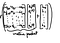Vectorization

We organize all of the activations from one layer into a column as a vector and then organize all of the weights as a matrix where each row of that matrix (vectorization) corresponds to the connections between one layer and a particular neuron in the next layer. This means that taking the weighted sum of the activations in the first layer according to these weights corresponds to one of the terms in the matrix-vector product.
We also organize all the biases into a vector and add the entire vector to the previous matrix-vector product and then rap a sigmoid around the outside the final product. And this now represents that the sigmoid function is applied to each specific component of the resulting vector inside the sigmoid function. So now we have a neat little expression to show the and full transition of activations from one layer to the next.

This makes the code a lot simpler and a lot faster since many libraries optimize the matrix multiplications.

We know that the gradient of a function gives us the direction of steepest ascent, where the positive gradient which direction should you step to increase the function most quickly and taking the negative of that gradient gives you the direction to step that decreases the function most quickly and the length of this gradient vector is an indication for just how steep that slope is. This vector tells us what the downhill direction is or the minimum is and how steep it is. Now computing this gradient direction and then taking a small step downhill and repeating this over and over gives us a minimum value for the cost function we were talking earlier about. The negative gradient of the cost function is just a vector or direction that tells us which nudges to all of those weights and biases is going to cause the most rapid decrease to the cost function and now when the slope is flattening out towards the minimum our steps get smaller and smaller and that kind of helps you from overshooting.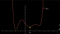This graph shows the various slopes and the paths the cost function will need to travel in order to minimize itself

Here changing the weights and biases to decrease the cost function means making the output of the network on each piece of training data look less like a random array of ten values and more like an actual decision that we want our network to make.
Again remember that this cost function involves an average over all of the training data. So minimizing it means it’s better performance on all of those data as a whole. The algorithm for computing this gradient efficiently is called back propagation and this process of repeatedly nudging the weights and biases by some multiple of the negative gradient is called gradient descent.

The weight, the previous activation, and the bias altogether are used to compute z, which in turn computes a after passing the z through an activation function and finally, along with the actual output y we compute the cost. Now we need to determine how sensitive the cost function is to small changes in our weight w^(L)or what’s the derivative of C with respect to w^(L). The “∂w” term means some tiny nudge to w and the “∂C” term refers to whatever the resulting nudge to the cost is. A slight change to w^(L) causes some change to z^(L) which in turn causes some change to a^(L), which directly influences the cost. So we divide this up by first looking at the ratio of a change to z^(L) to the change in w^(L) which is the derivative of z^(L) with respect to w^(L). Similarly, we then consider the ratio of a change to a^(L) to the change in z^(L) that caused it as well as the ratio between the final change to C and this intermediate change to a^(L). And this whole process is the chain rule, where multiplying together these three ratios gives us the sensitivity of C to tiny changes in w^(L). The derivative of C with respect to a^(L) is 2(a^(L) — y). The derivative of a^(L) with respect to z^(L) is just the derivative of the sigmoid function. And the derivative of z^(L) with respect to w^(L) comes out just to be a^(L-1). In the case of the last derivative(a^(L-1)), the amount that a small change to this weight influences the last layer depends on how strong the previous neuron is.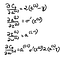Derivative w.r.t weights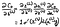Derivative w.r.t biases

The full cost function involves averaging together all those costs across many training examples, its derivative requires averaging this expression that we found overall training examples. And this is the gradient vector, which itself is built up from the partial derivatives of the cost function with respect to all those weights and biases.

Now we can just keep iterating the chain rule backward to see how sensitive is the cost function is to our previous weights and biases from the previous layers.

Rather than the activation of a given layer simply being a^(L), it’s also going to have a subscript indicating which neuron of that layer it is. Now let’s use the letter k to index the layer (L-1), and j to index the layer (L). For the cost we take a sum over (a_j^(L) - y_j)^2. Let’s call the weight of the edge connecting this k-th neuron to the j-th neuron w_{jk}^(L). The activation of the last layer is just your special function, like the sigmoid, applied to z. The chain-rule derivative expression describes how sensitive the cost is to a specific weight. What changes here is the derivative of the cost with respect to one of the activations in the layer (L-1), the neuron influences the cost function through multiple paths, i.e. it influences a_0^(L), which plays a role in the cost function, but it also has an influence on a_1^(L), which also plays a role in the cost function and we add those up. Now once we know how sensitive the cost function is to the activations in this second to the last layer, we can just repeat the process for all the weights and biases feeding into that layer. And finally after some considerable number of training steps(or epochs) we will have the desired accuracy in recognizing the digits or predicting a good apple.

# Why do we need neural networks at all?

Well as humans we are familiar and comfortable with all types of data which may be in the form of tables(structured) or images, speech, etc.(unstructured). But it has been much harder for computers to make sense of unstructured data as compared to structured data. And so this is one of the important reasons for the rise of neural networks which made the computers much better at interpreting unstructured data as well compared to just a few years ago. And this creates opportunities for many new applications that use speech recognition, image recognition, natural language processing on text, etc.
Another reason lies in the fact now-a-days data is generated in bulk which has eventually led to the Big Data era. So now our traditional machine learning algorithms have failed to show any further improvement with much larger amounts of data. So there come the neural networks to the rescue as it has the capability to learn from its own, unlike the traditional Machine learning algorithms which rely totally on the data. We just need a network with a lot of hidden units, a lot of parameters a lot of connections, as well as some considerable amount of the data to get better performance in the neural networks.

## More from Tanisha Banik

Data Science Enthusiast